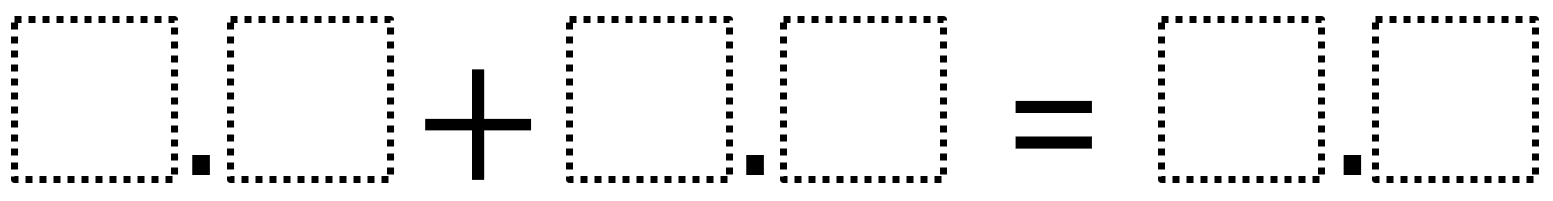# Decimal Addition 3

Directions: Use the digits 1 to 9, at most one time each, to make a sum with the greatest possible value.### Hint

How do you place digits so the sum is less than ten?

The least possible value is 1.2 and you can get it using many combinations of digits including:
5.2 + 4.6 = 9.8
3.1 + 6.7 = 9.8
2.5 + 7.3 = 9.8

Source: Owen Kaplinsky and Robert Kaplinsky

## Equivalent Expressions with Fractions

Directions: Using the digits 0 to 9 at most one time each and choosing either …

1.The ‘least possible value is 1.2’ and you can get it using many combinations of digits including:
5.2 + 4.6 = 9.8
3.1 + 6.7 = 9.8
2.5 + 7.3 = 9.8

I think this should read:
The greatest possible value is 9.8 and you can get it using many combinations of digits including:
5.2 + 4.6 = 9.8
3.1 + 6.7 = 9.8
2.5 + 7.3 = 9.8

•I agree!

2.Other solutions:

5.6 + 4.2 = 9.8
6.1 + 3.7 = 9.8
4.7 + 5.1 = 9.8
5.7 + 4.1 = 9.8
2.3 + 7.5 = 9.8

3.I thought you were suppose to add 1.9 +1.9 and i got 3.8.

This is so hard!!!!!

4.ig ot 6.7 + 3.1

5.The least possible value is 1.2 and you can get it using many combinations of digits including:
5.2 + 4.6 = 9.8
3.1 + 6.7 = 9.8
2.5 + 7.3 = 9.8

6.6.8+2.8=9.6
7.8+1.8=9.8

•1.7+8.1=9.8
The least possible value is 1.2

7.6.8+3.1=9.9

8.1.2+3.4=4.6

9.5.2+4.6=9.8